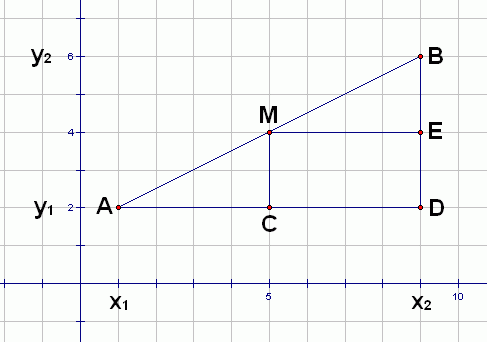#The Midpoint Formula

Want practice with preliminary concepts first?

THE MIDPOINT FORMULA
The midpoint of the line segment between points $\,(x_1,y_1)\,$ and $\,(x_2,y_2)\,$ is given by the Midpoint Formula: $$\cssId{s6}{\left( \frac{x_1+x_2}2,\frac{y_1+y_2}2 \right)}$$

Here, $\,x_1\,$ (read as ‘$\,x\,$ sub $\,1\,$’) denotes the $\,x$-value of the first point, and $\,y_1\,$ (read as ‘$\,y\,$ sub $\,1\,$’) denotes the $\,y$-value of the first point. Similarly, $\,x_2\,$ and $\,y_2\,$ denote the $\,x$-value and $\,y$-value of the second point.

Thus, to find the location that is exactly halfway between two points, you average the $x$-values, and average the $y$-values.

The Midpoint Formula follows easily from the following observations:

• Referring to the sketch below, $\Delta ABD\,$ is similar to $\,\Delta AMC\,.$ That is, these two triangles have the same angles. Why? They both share angle $\,A\,,$ and they both have a right angle. Since all the angles in a triangle sum to $\,180^\circ\,,$ the third angles must also be the same.• Similarity gives us what we need! It tells us that $\Delta ABD\,$ and $\Delta AMC\,$ have exactly the same shapes—they're just different sizes. Since $\,\overline{AM}\,$ is exactly half of $\,\overline{AB}\,,$ $\,\overline{AC}\,$ must be exactly half of $\,\overline{AD}\,.$ Thus, $\,C\,$ is the midpoint between $\,A\,$ and $\,D\,$ (which can be found by averaging $\,x_1\,$ and $\,x_2\,$).
• Use a similar argument to show that $\,\overline{DE}\,$ (which has the same length as $\,\overline{CM}\,$) is exactly half of $\,\overline{DB}\,.$

## Examples

Question: Find the midpoint of the line segment between $\,(1,-3)\,$ and $\,(-2,5)\,.$
Solution: $$\cssId{s33}{\left( \frac{1+(-2)}2, \frac{-3 + 5}2 \right)} \cssId{s34}{= \left(-\frac12,1\right)}$$
Question: Suppose that $\,(2,3)\,$ is exactly halfway between $\,(-1,5)\,$ and $\,(x,y)\,.$ Find $\,x\,$ and $\,y\,.$
Solution: Rephrasing, $\,(2,3)\,$ is the midpoint of the segment with endpoints $\,(-1,5)\,$ and $\,(x,y)\,.$ Thus:
 \displaystyle \begin{align} &\cssId{s41}{(2,3)}\cr &\quad \cssId{s42}{= \left(\frac{-1+x}2,\frac{5+y}2\right)} \end{align} use the Midpoint Formula \displaystyle \begin{align} &\cssId{s44}{2 = \frac{-1+x}2}\cr &\quad \cssId{s45}{\text{and }}\ \cssId{s46}{3 = \frac{5+y}2} \end{align} for ordered pairs to be equal, the first coordinates must be equal and the second coordinates must be equal \displaystyle \begin{align} &\cssId{s50}{4 = -1 + x}\cr &\quad \cssId{s51}{\text{and }} \cssId{s52}{6 = 5 + y} \end{align} clear fractions (multiply both sides of both equations by $\,2$) $5 = x\$ and $\ 1 = y$ finish solving each equation $x = 5\$ and $\ y = 1$ write your solutions in the conventional way